GATE  >  Computer Science And Information Technology - CS 2016 GATE Paper (Practice Test)

# Computer Science And Information Technology - CS 2016 GATE Paper (Practice Test) - GATE

Test Description

## 65 Questions MCQ Test GATE Past Year Papers for Practice (All Branches) - Computer Science And Information Technology - CS 2016 GATE Paper (Practice Test)

Computer Science And Information Technology - CS 2016 GATE Paper (Practice Test) for GATE 2023 is part of GATE Past Year Papers for Practice (All Branches) preparation. The Computer Science And Information Technology - CS 2016 GATE Paper (Practice Test) questions and answers have been prepared according to the GATE exam syllabus.The Computer Science And Information Technology - CS 2016 GATE Paper (Practice Test) MCQs are made for GATE 2023 Exam. Find important definitions, questions, notes, meanings, examples, exercises, MCQs and online tests for Computer Science And Information Technology - CS 2016 GATE Paper (Practice Test) below.
Solutions of Computer Science And Information Technology - CS 2016 GATE Paper (Practice Test) questions in English are available as part of our GATE Past Year Papers for Practice (All Branches) for GATE & Computer Science And Information Technology - CS 2016 GATE Paper (Practice Test) solutions in Hindi for GATE Past Year Papers for Practice (All Branches) course. Download more important topics, notes, lectures and mock test series for GATE Exam by signing up for free. Attempt Computer Science And Information Technology - CS 2016 GATE Paper (Practice Test) | 65 questions in 180 minutes | Mock test for GATE preparation | Free important questions MCQ to study GATE Past Year Papers for Practice (All Branches) for GATE Exam | Download free PDF with solutions
 1 Crore+ students have signed up on EduRev. Have you?
Computer Science And Information Technology - CS 2016 GATE Paper (Practice Test) - Question 1

### Q. 1 – Q. 5 carry one mark each. Q. Out of the following four sentences, select the most suitable sentence with respect to grammar and usage.

Detailed Solution for Computer Science And Information Technology - CS 2016 GATE Paper (Practice Test) - Question 1

‘until’ itself is negative so it can’t take one more negative i.e., ‘does not’. Hence, Option (D) is the

Computer Science And Information Technology - CS 2016 GATE Paper (Practice Test) - Question 2

### A rewording of something written or spoken is a ______________.

Detailed Solution for Computer Science And Information Technology - CS 2016 GATE Paper (Practice Test) - Question 2

‘paraphrase’ means a restatement of a text, passage or a rewording of something written or spoken.

Computer Science And Information Technology - CS 2016 GATE Paper (Practice Test) - Question 3

### Archimedes said, “Give me a lever long enough and a fulcrum on which to place it, and I will move the world.” The sentence above is an example of a ___________ statement

Detailed Solution for Computer Science And Information Technology - CS 2016 GATE Paper (Practice Test) - Question 3

‘figurative’ means representing by a figure or resemblance or expressing one thing in terms normally denoting another with which it may be regarded as analogous.

Computer Science And Information Technology - CS 2016 GATE Paper (Practice Test) - Question 4

If ‘relftaga’ means carefree, ‘otaga’ means careful and ‘fertaga’ means careless, which of the following could mean ‘aftercare’?

Detailed Solution for Computer Science And Information Technology - CS 2016 GATE Paper (Practice Test) - Question 4

From given codes
relftaga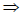carefree
Otagacareful
Fertagacareless
From these codes, clearly known that “care” means “taga,” from given alternatives, option ‘C’ is correct.

Computer Science And Information Technology - CS 2016 GATE Paper (Practice Test) - Question 5

A cube is built using 64 cubic blocks of side one unit. After it is built, one cubic block is removed from every corner of the cube. The resulting surface area of the body (in square units) after the removal is __________.

Detailed Solution for Computer Science And Information Technology - CS 2016 GATE Paper (Practice Test) - Question 5

From given data, 64 cubic blocks of one unit
Sizes are formed No of faces of the
Cube is ‘6’ No of corners of the
Cube is ‘8’ After removing one
Cubic block from Each corner,
The resulting surface area of the body = 6 x (4) = 96 sq. Units.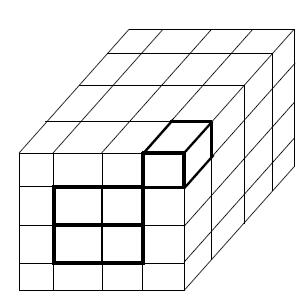Computer Science And Information Technology - CS 2016 GATE Paper (Practice Test) - Question 6

Q. 6 – Q. 10 carry two marks each.

Q.

A shaving set company sells 4 different types of razors, Elegance, Smooth, Soft and Executive. Elegance sells at Rs. 48, Smooth at Rs. 63, Soft at Rs. 78 and Executive at Rs. 173 per piece. The table below shows the numbers of each razor sold in each quarter of a year.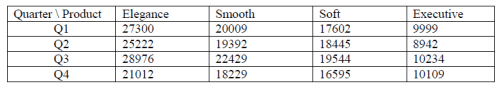Which product contributes the greatest fraction to the revenue of the company in that year?

Detailed Solution for Computer Science And Information Technology - CS 2016 GATE Paper (Practice Test) - Question 6

Total No. of razors Elegance type from all four quarters = 27300 + 25222 + 28976 + 201012
= 10,2510
Total No. of razors of Smooth type from all four quarters = 20009 + 19392 + 22429 + 18229
= 8,0059
Total No. of razors of Soft type from all four Quarters = 17602 + 18445 + 19544 + 16595
= 7,2186
Total No. of razors of Executive type from all four Quarters = 9999 + 8942 + 10234 + 10109
= 3,9286
The revenue of the company in that year of 4 different types of razors
Elegance = 10,2510 x 48 = 49,20,480
Smooth = 8,0059 x 63 = 50,43,717
Soft = 7,2186 x 78 = 56,30,508
Executive = 3,9284 x 193 = 67,96,132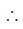The Executive of razors contributes the greatest revenue of the company in that year.

Computer Science And Information Technology - CS 2016 GATE Paper (Practice Test) - Question 7

Indian currency notes show the denomination indicated in at least seventeen languages. If this is not an indication of the nation’s diversity, nothing else is.

Which of the following can be logically inferred from the above sentences?

Detailed Solution for Computer Science And Information Technology - CS 2016 GATE Paper (Practice Test) - Question 7

If seventeen languages were not an indication of the nation’s diversity, nothing else is. If nothing else is so the best inference is option ‘D’.

Computer Science And Information Technology - CS 2016 GATE Paper (Practice Test) - Question 8

Consider the following statements relating to the level of poker play of four players P, Q, R and S.
I. P always beats Q
II. R always beats S
III. S loses to P only sometimes
IV. R always loses to Q

Which of the following can be logically inferred from the above statements?

(i) P is likely to beat all the three other players
(ii) S is the absolute worst player in the set

Detailed Solution for Computer Science And Information Technology - CS 2016 GATE Paper (Practice Test) - Question 8

From the given data
Player P > Player Q
Player R > Player S
Player S < Player P (sometimes only)
Player R < Player QPlayer ‘P’ > Player ‘Q’ > Player ‘R’ > Player ‘S’Logically, the statement (i) is definitely true, but statement (ii) is notOption ‘A’ is true

Computer Science And Information Technology - CS 2016 GATE Paper (Practice Test) - Question 9

If f(x) = 2x7+3x−5, which of the following is a factor of f(x)?

Detailed Solution for Computer Science And Information Technology - CS 2016 GATE Paper (Practice Test) - Question 9

f (x) = 2x7 + 3x - 5 for x = 1 the equation is satisfied. The factor is (x–1).

Computer Science And Information Technology - CS 2016 GATE Paper (Practice Test) - Question 10

In a process, the number of cycles to failure decreases exponentially with an increase in load. At a load of 80 units, it takes 100 cycles for failure. When the load is halved, it takes 10000 cycles for failure. The load for which the failure will happen in 5000 cycles is ________.

Detailed Solution for Computer Science And Information Technology - CS 2016 GATE Paper (Practice Test) - Question 10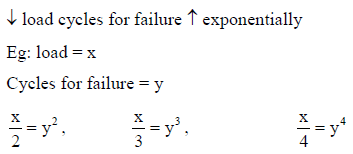Option (A)
Load = 40units, it says that, the load is halved, it takes 10,000 cycles for failure. 80/2 =(100)

40 units = 10,000 cycles, it is not

Option (D)
Load = 92.02 units means it is more than 80 units, so it is not.
Option (C)
3/4(80 units) = 60.01 units
From the given relation
3/4(80 units) = (10)4/3 = (100)1 x (100)1/3 = 100 x 4.64 = 464
It is notOption (B) only possibleAt the load of 46.02 units, the failure will happen in 5000 cycles.

*Answer can only contain numeric values
Computer Science And Information Technology - CS 2016 GATE Paper (Practice Test) - Question 11

Q.11-Q.35carryonemarkeach.

Q.

Let p,q, r; s represent thefollowing propositions.
p: x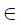{8,9,10,11,12}
q: x is acompositenumber
r: x is aperfectsquare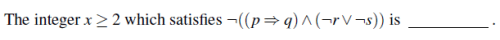Detailed Solution for Computer Science And Information Technology - CS 2016 GATE Paper (Practice Test) - Question 11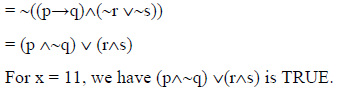Computer Science And Information Technology - CS 2016 GATE Paper (Practice Test) - Question 12

Let an be thenumberof n-bit strings that do NOT contain two consecutive 1s.Which one of
the following is the recurrence relation for an?

Detailed Solution for Computer Science And Information Technology - CS 2016 GATE Paper (Practice Test) - Question 12

Let an denote the number of n-bit strings that do not contain two consecutive 1’s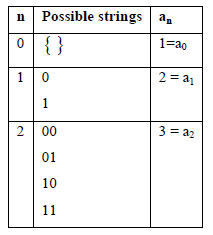By observing above analysis, we can write an = an–1+ an–2

(OR)
Case 1: If the first bit is zero, then the remaining bits we can choose in an–1 ways.
Case 2: If the first bit is one, then the second bit is 0 and the remaining bits we can choose in an–2
ways.
The recurrence relation is an = an–1+ an–2

*Answer can only contain numeric values
Computer Science And Information Technology - CS 2016 GATE Paper (Practice Test) - Question 13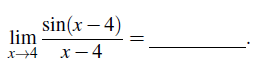Detailed Solution for Computer Science And Information Technology - CS 2016 GATE Paper (Practice Test) - Question 13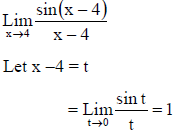(OR)

The given limit is in 0/0 form. Applying L. Hospital’s rule, we get
The limiting value = 1

*Answer can only contain numeric values
Computer Science And Information Technology - CS 2016 GATE Paper (Practice Test) - Question 14

A probabilitydensity function on the interval [a,1] is given by1/x2 and outside this interval the value of the function is zero.The value of a is _____________.

Detailed Solution for Computer Science And Information Technology - CS 2016 GATE Paper (Practice Test) - Question 14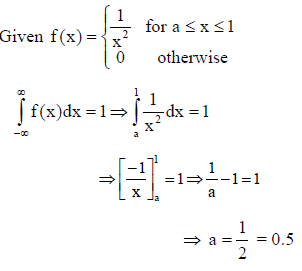*Answer can only contain numeric values
Computer Science And Information Technology - CS 2016 GATE Paper (Practice Test) - Question 15

Two eigenvalues of a 3 x 3 real matrix P are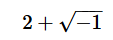and 3 .The determinant of P is ______________.

Detailed Solution for Computer Science And Information Technology - CS 2016 GATE Paper (Practice Test) - Question 15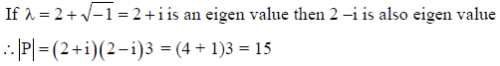(OR)
Complex roots of a polynomial equation always occur in pairs.
If (2 + i) is an eigen value then (2 – i) is also an eigen value of P.
Determinant of P = (2 + i) (2 – i) 3 = 15

Computer Science And Information Technology - CS 2016 GATE Paper (Practice Test) - Question 16

In the following truth table, V = 1 if and only if the input is valid.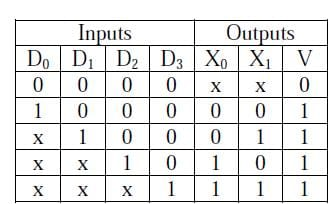Detailed Solution for Computer Science And Information Technology - CS 2016 GATE Paper (Practice Test) - Question 16

Since there are more than one outputs and number of outputs is less than inputs, it is a Priority encoder V=1 when input is valid and for priority encoder it checks first high bit encountered. Except all are having at least one bit high and ‘x’ represents the “don’t care” as we have found a high bit already. So answer is (A).

*Answer can only contain numeric values
Computer Science And Information Technology - CS 2016 GATE Paper (Practice Test) - Question 17

The 16-bit 2’s complement representation of an integer is1111 1111 1111 0101; its decimal representation is  ______________.

Detailed Solution for Computer Science And Information Technology - CS 2016 GATE Paper (Practice Test) - Question 17

Integer size is 16 bit, already it is given in it’s 2’s complement notation; So, when it is 2’s
complemented once again it gives value. So answer is –11.

*Answer can only contain numeric values
Computer Science And Information Technology - CS 2016 GATE Paper (Practice Test) - Question 18

We want to design asynchronous counter that counts the sequence 0-1-0-2-0-3 and then repeats. The minimum number of J-K flip-flops required to implement this counter is ____________.

Detailed Solution for Computer Science And Information Technology - CS 2016 GATE Paper (Practice Test) - Question 18

In the given first loop of states, zero has repeated 3 times. So, minimum 4 number of Flip-flops are needed.

*Answer can only contain numeric values
Computer Science And Information Technology - CS 2016 GATE Paper (Practice Test) - Question 19

A processor can support a maximum memory of 4GB,where the memory is word - addressable
(a word consists of two bytes).The size of the address bus of the processor is at least _________ bits.

Detailed Solution for Computer Science And Information Technology - CS 2016 GATE Paper (Practice Test) - Question 19

Word size = 16 bit,
so memory size = 231 words.31 address bits are needed.

Computer Science And Information Technology - CS 2016 GATE Paper (Practice Test) - Question 20

A queue is implemented using an array such that ENQUEUE and DEQUEUE operations are
performed efficiently. Which one of the following statements is CORRECT (n refers to the
number of items in the queue)?

*Answer can only contain numeric values
Computer Science And Information Technology - CS 2016 GATE Paper (Practice Test) - Question 21

Consider the following directed graph: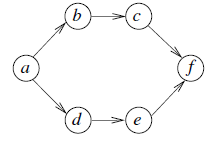The number of different topological orderings of the vertices of the graph is _____________ .

Detailed Solution for Computer Science And Information Technology - CS 2016 GATE Paper (Practice Test) - Question 21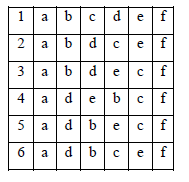Computer Science And Information Technology - CS 2016 GATE Paper (Practice Test) - Question 22

Consider the following C program.

void f(int,short);
void main()
{
int i=100;
short s=12;
short *p=&s;
__________ ;//calltof()
}

Which one of the following expressions,when placed in the blank above, will NOT result in
a type checking error?

Detailed Solution for Computer Science And Information Technology - CS 2016 GATE Paper (Practice Test) - Question 22

i is integer and *p is value of a pointer to short.

1) Option 1 is wrong because we are passing “*S” as second argument check that S is not a pointer variable .So error
2) Second option is we are trying to store the value of f(i,s) into i but look at the function definition outside main it has no return type. It is simply void so that assignment is wrong. So error
3) Option 3 is wrong because of the same reason why option 1 is wrong
4) So option d is correct.

Computer Science And Information Technology - CS 2016 GATE Paper (Practice Test) - Question 23

The worst case running times of Insertion sort, Merge sort and Quick sort, respectively,are:

Computer Science And Information Technology - CS 2016 GATE Paper (Practice Test) - Question 24

Let G be aweighted connected undirected graph with distinct positive edge weights.If every edge weight is increased by the same value,then which of the following statements is/are TRUE?

P: Minimum spanning tree of G does notchange
Q: Shortest path between any pair of vertices does not change

*Answer can only contain numeric values
Computer Science And Information Technology - CS 2016 GATE Paper (Practice Test) - Question 25

Consider the follwing  C Program.

#include<stdio.h>
void mystery(int*ptra,int*ptrb){
int *temp;
temp =ptrb;
ptrb =ptra;
ptra =temp;
}
int main(){
int a=2016,b=0,c=4,d=42;
mystery(&a, &b);
if (a<c)
mystery(&c, &a);
mystery(&a, &d);
printf("%d ", a);
}

The output of the program is _____________ .

Detailed Solution for Computer Science And Information Technology - CS 2016 GATE Paper (Practice Test) - Question 25

Whatever modifications are performed in mystery ( ) function, those modifications are not reflected
in main ( ) function so it will print 2016.

Computer Science And Information Technology - CS 2016 GATE Paper (Practice Test) - Question 26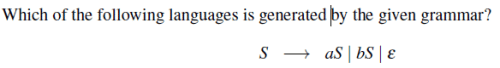Detailed Solution for Computer Science And Information Technology - CS 2016 GATE Paper (Practice Test) - Question 26

S → aS |bS |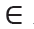S → (a + b)*
{a, b}* = (a + b)*

Computer Science And Information Technology - CS 2016 GATE Paper (Practice Test) - Question 27

Which of the following decision problems are undecidable?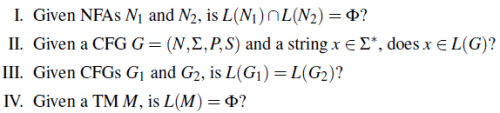Detailed Solution for Computer Science And Information Technology - CS 2016 GATE Paper (Practice Test) - Question 27

III. Equality of CFG is undecidable
IV. Emptiness of TM is undecidable

Computer Science And Information Technology - CS 2016 GATE Paper (Practice Test) - Question 28

Which one of the following regular expressions represents the language: the set of all binary strings having two consecutive 0s and two consecutive 1s?

*Answer can only contain numeric values
Computer Science And Information Technology - CS 2016 GATE Paper (Practice Test) - Question 29

Consider the following code segment.
x =u-t;
y =x*v;
x =y+w;
y =t-z;
y =x*y;
The minimum number of total variables required to convert the above code segment to static single assignment form is ______________ .

Computer Science And Information Technology - CS 2016 GATE Paper (Practice Test) - Question 30

What type of memory is volatile ?

Computer Science And Information Technology - CS 2016 GATE Paper (Practice Test) - Question 31

Which of the following is NOT a super key in a relational schema with attributes V,W, X, Y, Z and primarykey V Y?

Detailed Solution for Computer Science And Information Technology - CS 2016 GATE Paper (Practice Test) - Question 31

A superkey is one which contains a candidate key.

Computer Science And Information Technology - CS 2016 GATE Paper (Practice Test) - Question 32

Which one of the following is NOT a part of the ACID properties of database transactions?

Detailed Solution for Computer Science And Information Technology - CS 2016 GATE Paper (Practice Test) - Question 32

A: Atomicity
C: Consistency
I: Isolation
D: Durability

Computer Science And Information Technology - CS 2016 GATE Paper (Practice Test) - Question 33

A database of research articles in a journal uses the following schema.
(VOLUME, NUMBER, STARTPAGE, ENDPAGE, TITLE, YEAR, PRICE)
The primary key is(VOLUME, NUMBER, STARTPAGE, ENDPAGE) and the following functional dependenciesexist in the schema.

(VOLUME, NUMBER, STARTPAGE, ENDPAGE) → TITLE
(VOLUME, NUMBER)                                        → YEAR
(VOLUME, NUMBER, STARTPAGE, ENDPAGE) → PRICE
The database is redesigned to use the following schemas.
(VOLUME, NUMBER, STARTPAGE, ENDPAGE, TITLE, PRICE)
(VOLUME, NUMBER, YEAR)
Which is the weakest normal form that the new database satisfies,but the old one does not?

Detailed Solution for Computer Science And Information Technology - CS 2016 GATE Paper (Practice Test) - Question 33

(Volume, Number) → Year is a partial functional dependency. So, the given relation is in 1 NF but
not in 2 NF.

Computer Science And Information Technology - CS 2016 GATE Paper (Practice Test) - Question 34

Which one of the following protocols is NOT used to resolve one form of address to another one?

Detailed Solution for Computer Science And Information Technology - CS 2016 GATE Paper (Practice Test) - Question 34

DHCP is dynamic host configuration protocol: allocates one of the unused IP address

Computer Science And Information Technology - CS 2016 GATE Paper (Practice Test) - Question 35

Which of the following is/are example(s) of stateful application layer protocols?
(i) HTTP
(ii) FTP
(iii) TCP
(iv) POP3

Detailed Solution for Computer Science And Information Technology - CS 2016 GATE Paper (Practice Test) - Question 35

POP3 and FTP are stateful application layer protocols

*Answer can only contain numeric values
Computer Science And Information Technology - CS 2016 GATE Paper (Practice Test) - Question 36

Q.36-Q.65 carry two marks each.

Q.

The coefficient of x12 in (x3+x4+x5+x6+... )3 is ____________ .

Detailed Solution for Computer Science And Information Technology - CS 2016 GATE Paper (Practice Test) - Question 36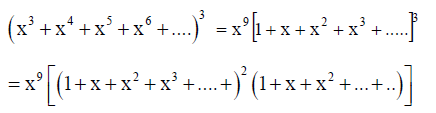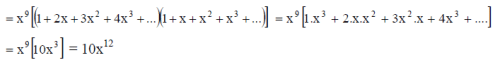(OR)*Answer can only contain numeric values
Computer Science And Information Technology - CS 2016 GATE Paper (Practice Test) - Question 37

Consider the recurrence relation a1 = 8, an = 6n2+2n+an-1. Let a99 = K x104. The value
of K is  ___________.

Detailed Solution for Computer Science And Information Technology - CS 2016 GATE Paper (Practice Test) - Question 37

The recurrence relation is an – an-1 = 6n2 + 2n
Complementary function = C1
Let particular solution = (An2 + Bn C)n
Substituting in the recurrence relation, and solving we get A = 2, B = 4 and C = 2.The solution is an = C1 + 2n3 + 4n2 + 2n
a1 = 88 = C1 + 8C1 = 0
a99 = 2{(993) + 2(992) + 99}
= 2{(100 – 1)3 + 2(100 – 1)2 + (100 – 1)}
= 104 (198)k = 198

*Answer can only contain numeric values
Computer Science And Information Technology - CS 2016 GATE Paper (Practice Test) - Question 38

A function f : N+ → N+, defined on the set of positive integers N+, satisfies the following
properties:
f (n) = f (n=2) if n is even
f (n) = f (n+5) if n is odd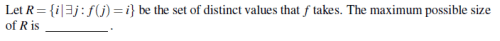Detailed Solution for Computer Science And Information Technology - CS 2016 GATE Paper (Practice Test) - Question 38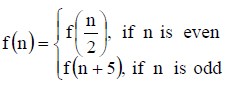Using the definition of the function we can show that
f(1) = f(2) = f(3) = f(4) = f(6) = f(7) = f(8) = f(9) …
and f(5) = f(10) = f(15) = f(20) = …..The range of f(n) contain two distinct elements.

*Answer can only contain numeric values
Computer Science And Information Technology - CS 2016 GATE Paper (Practice Test) - Question 39

Consider the following experiment.
Step 1. Flip a fair coin twice.
Step 2. If the outcomes are(TAILS, HEADS) then output Y and stop.
stop.
Step 4. If the outcomes are(TAILS, TAILS), then go to Step1.
The probability that the output of the experimentis Y is (up to two decimal places) ____________.

Detailed Solution for Computer Science And Information Technology - CS 2016 GATE Paper (Practice Test) - Question 39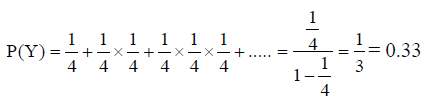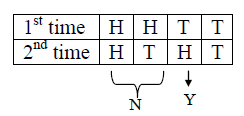Computer Science And Information Technology - CS 2016 GATE Paper (Practice Test) - Question 40

Consider the two cascaded 2 - to - 1 multiplexers as shown in the figure.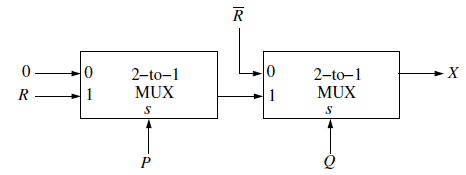Detailed Solution for Computer Science And Information Technology - CS 2016 GATE Paper (Practice Test) - Question 40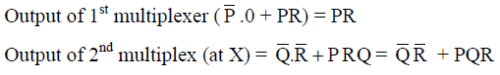*Answer can only contain numeric values
Computer Science And Information Technology - CS 2016 GATE Paper (Practice Test) - Question 41

The size of the data count register of a DMA controller is 16 bits. The processor needs to transfer a file of 29,154 kilobytes from disk to main memory. The memory is byte addressable. The minimum number of times the DMA controller needs to get the c

Detailed Solution for Computer Science And Information Technology - CS 2016 GATE Paper (Practice Test) - Question 41

Size of data count register of the DMA controller = 16 bits
Data that can be transferred in one go = 216 bytes = 64 kilobytes
File size to be transferred = 29154 kilobytes
So, number of times the DMA controller needs to get the control of the system bus from the processor to transfer the file from the disk to main memory = ceil(29154/64) = 456

*Answer can only contain numeric values
Computer Science And Information Technology - CS 2016 GATE Paper (Practice Test) - Question 42

The stage delays in a 4 - stage pipeline are 800,500,400 and 300 picoseconds.The first stage (with delay 800 picoseconds) is replaced with a functionally equivalent design involving two stages with respective delays 600 and 350 picoseconds.The through put increase of the pipeline is  ____________percent.

Detailed Solution for Computer Science And Information Technology - CS 2016 GATE Paper (Practice Test) - Question 42

Old pipeline maximum delay = 800 ns
New pipeline maximum delay = 600 ns
800 : 600 = 4:3
Increasing throughput= (4-3)/3= 33.3%

Computer Science And Information Technology - CS 2016 GATE Paper (Practice Test) - Question 43

Detailed Solution for Computer Science And Information Technology - CS 2016 GATE Paper (Practice Test) - Question 43

Size of the integer = n bit and maximum number of inputs to the gate is two. So, time is
depending on size.

Computer Science And Information Technology - CS 2016 GATE Paper (Practice Test) - Question 44

The following function computes the maximum value contained in an integer array p[ ] of size

n (n>=1).
int max(int*p,intn){
int a=0,b=n-1;
while (__________){
if (p[a]<=p[b]){a=a+1;}
else {b=b-1;}
}
return p[a];
}
The missing loop condition is

Detailed Solution for Computer Science And Information Technology - CS 2016 GATE Paper (Practice Test) - Question 44

If b! = a we get maximum element of an integer

Computer Science And Information Technology - CS 2016 GATE Paper (Practice Test) - Question 45

What will be the output of the following C program ?

void count(intn){
static intd=1;
printf("%d ",n);
printf("%d ",d);
d++;
if(n>1) count(n-1);
printf("%d ",d);
}
void main(){
count(3);
}

Detailed Solution for Computer Science And Information Technology - CS 2016 GATE Paper (Practice Test) - Question 45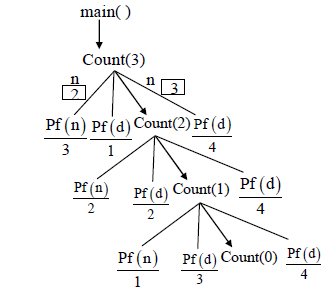Output = 3, 1, 2, 2, 1, 3, 4, 4, 4

Computer Science And Information Technology - CS 2016 GATE Paper (Practice Test) - Question 46

What will be the output of the following pseudo-code when parameters are passed by reference
and dynamic scoping is assumed?
a=3;
void n(x){x=x*a;print(x);}
void m(y){a=1;a=y-a;n(a);print(a);}
void main(){m(a);}

Detailed Solution for Computer Science And Information Technology - CS 2016 GATE Paper (Practice Test) - Question 46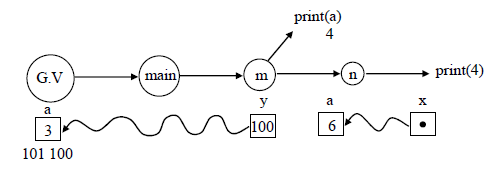Computer Science And Information Technology - CS 2016 GATE Paper (Practice Test) - Question 47

An operator delete(i)delete(i) for a binary heap data structure is to be designed to delete the item in the ii-th  node. Assume that the heap is implemented in an array and ii refers to the ii-th index of the array. If the heap tree has depth dd (number of edges on the path from the root to the farthest leaf ), then what is the time complexity to re-fix the heap efficiently after the removal of the element?

*Answer can only contain numeric values
Computer Science And Information Technology - CS 2016 GATE Paper (Practice Test) - Question 48

Consider the weighted undirected graph with 4 vertices,where the weight of edge {i; j} is
given by the entry Wi j in the matrix W.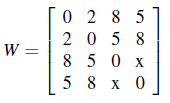The largest possible integer value of x, for which at least one shortest path between some pair
of vertices will contain the edge with weight x is .

*Answer can only contain numeric values
Computer Science And Information Technology - CS 2016 GATE Paper (Practice Test) - Question 49

Let G be acomplete undirected graph on 4 vertices,having 6 edges with weights being1,2, 3, 4,5,and 6.The maximum possible weight that a minimum weight spanning treeof G can have is  _________.

Computer Science And Information Technology - CS 2016 GATE Paper (Practice Test) - Question 50

G = (V;E) is an undirected simple graph in which each edge has a distinct weight,and e is a particular edgeof G. Which of the following statements about the minimum spanning trees (MSTs) of G is/are TRUE?

I. If e is the lightest edge of some cycle in G, then every MST of G includes e
II. If e is the heaviest edge of some cycle in G, then everyMST of G excludes e

Detailed Solution for Computer Science And Information Technology - CS 2016 GATE Paper (Practice Test) - Question 50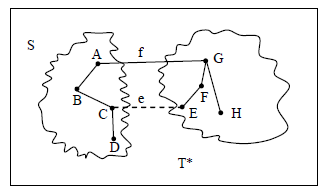I. TRUE
Suppose ‘e’ does not belong to T*
Adding ‘e’ to T* creates a(unique) cycle ‘C’ in T*
Some other edge in cycle ‘C’, say ‘f’ has exactly one end point in ‘s’
T = T* ?{e}–f is also spanning Tree and since Ce < Cf
So,cost(T) < cost(T*)
Which is contridicts minimality of T*.

II. TRUE
Suppose ‘f’ belongs to T*
Deleting ‘f’ from T* disconnects T* and let ‘s’ be one side of cut.

Some other edge in cycle ‘C’ say ‘e’ has exactly one end point in S
T = T*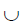{e} – {f} is also a spanning Tree.
Since Ce < Cf so cost(T) < cost(T*)
Which is contradictory to minimality of T*

*Answer can only contain numeric values
Computer Science And Information Technology - CS 2016 GATE Paper (Practice Test) - Question 51

Let Q denote a queue containing sixteen numbers and S be an empty stack. Head (Q) returns the element at the head of the queue Q without removingitfrom Q. Similarly Top(S) returns the element at the top of S without removing it from S. Consider the algorithm given below.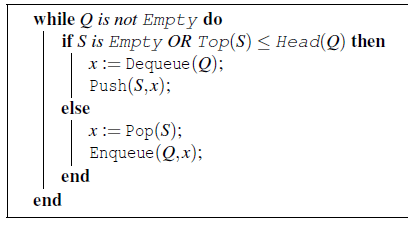The maximum possible number of iterations of the while loop in the algorithm is ________.
.

Detailed Solution for Computer Science And Information Technology - CS 2016 GATE Paper (Practice Test) - Question 51

Trying with queue elements, say n = 1, 2, 3, then maximum number of iterations are noticed as n2.
Here n = 16, So answer will be 256.

Computer Science And Information Technology - CS 2016 GATE Paper (Practice Test) - Question 52

Consider the following context - free grammars: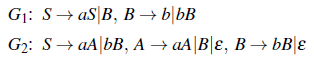Which one of the following pairs of languages is generated by G1 and G2, respectively?

Detailed Solution for Computer Science And Information Technology - CS 2016 GATE Paper (Practice Test) - Question 52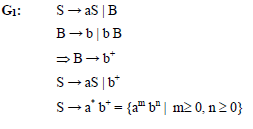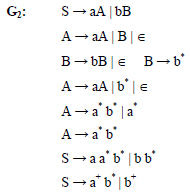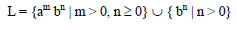Computer Science And Information Technology - CS 2016 GATE Paper (Practice Test) - Question 53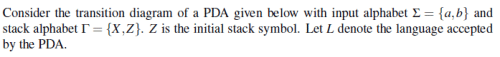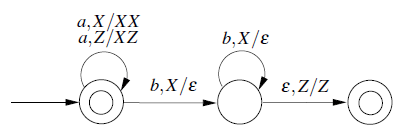Which one of the following is TRUE?

Detailed Solution for Computer Science And Information Technology - CS 2016 GATE Paper (Practice Test) - Question 53

L = {an | n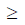0}{an bn | n0} and
L is DCFL

Computer Science And Information Technology - CS 2016 GATE Paper (Practice Test) - Question 54

Let X be are cursive language and Y be are cursively enumerable but not recursive language.
Let W and Z be two languages such that Y reduces to W, and Z reduces to X (reduction means
the standard many-one reduction).Which one of the following statements is TRUE?

Detailed Solution for Computer Science And Information Technology - CS 2016 GATE Paper (Practice Test) - Question 54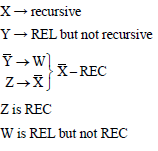*Answer can only contain numeric values
Computer Science And Information Technology - CS 2016 GATE Paper (Practice Test) - Question 55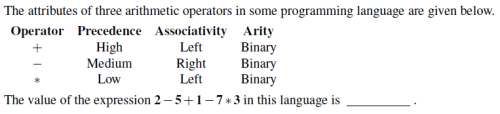Detailed Solution for Computer Science And Information Technology - CS 2016 GATE Paper (Practice Test) - Question 55

2–5+1–7*3
2-6–7*3
2-(–1)*3
3*3
9

Computer Science And Information Technology - CS 2016 GATE Paper (Practice Test) - Question 56

Consider the following Syntax Directed Translation Scheme (SDTS), with non-terminals {S, A} and terminals {a, b}.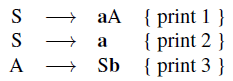Using the above SDTS, the output printed by a bottom-up parser,for the input aab is:

Detailed Solution for Computer Science And Information Technology - CS 2016 GATE Paper (Practice Test) - Question 56

S → aA {print 1}
S → a {print 2}
S → Sb {print 3}
Input: aab*Answer can only contain numeric values
Computer Science And Information Technology - CS 2016 GATE Paper (Practice Test) - Question 57

Consider a computer system with 40-bit virtual addressing and page size of sixteen kilobytes. If the computer system has a one-level page table perprocess and each page table entry requires48 bits,then the size of the per-process page table is megabytes.

*Answer can only contain numeric values
Computer Science And Information Technology - CS 2016 GATE Paper (Practice Test) - Question 58

Consider a disk queue with requests for I/O to blocks on cylinders 47,38,121,191,87,11, 92, 10.The C-LOOK scheduling algorithm is used.The head is initially at cylinder number 63, moving towards larger cylinder numbers on its servicing pass.The cylinders are numbered from 0 to199.The total head movement (in number of cylinders) incurred while servicing these requestsis .

Computer Science And Information Technology - CS 2016 GATE Paper (Practice Test) - Question 59

Consider a computer system with ten physical page frames.The system is provided with an access sequence (a1;a2; :::;a20;a1;a2; :::;a20), where each ai is a distinct virtual page number.The difference in the number of page faults between the last-in-first-outpage replacement policy and the optimal page replacement policyis .

Computer Science And Information Technology - CS 2016 GATE Paper (Practice Test) - Question 60

Consider the following proposed solution for the critical section problem.There are n
processes: P0 ...Pn-1. In the code, function pmax returns an integer not smaller than any
of its arguments.For all i, t[i] is initialized to zero.
Code for Pi:

do {
c[i]=1; t[i]=pmax(t,: ::,t[n-1])+1; c[i]=0;
for every j 6= i in{0,: ::,n-1} {
while (c[j]);
while (t[j]!=0 && t[j]<=t[i]);
}
Critical Section;
t[i]=0;
Remainder Section;
} while(true);

Which one of the following is TRUE about the above solution?

Computer Science And Information Technology - CS 2016 GATE Paper (Practice Test) - Question 61

Consider the following two phase locking protocol. Suppose a transaction T accesses (for read or write operations), a certain set of objects {O1,…,Ok}. This is done in the following manner:

Step 1. T acquires exclusive locks to O1, . . . , Ok in increasing order of their addresses.
Step 2. The required operations are performed.
Step 3. All locks are released.

This protocol will

Computer Science And Information Technology - CS 2016 GATE Paper (Practice Test) - Question 62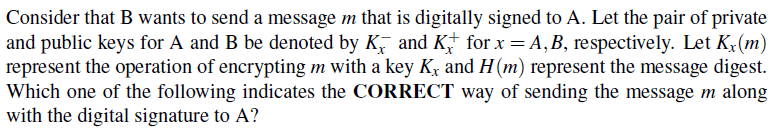*Answer can only contain numeric values
Computer Science And Information Technology - CS 2016 GATE Paper (Practice Test) - Question 63

An IP data gram of size 1000 bytes arrives at a router.The router has to forward this packet on
a link whose MTU(maximum transmission unit) is100bytes. Assume that the size of the IP
The number of fragments that the IP data gram will be divided into for transmissionis___________.

Detailed Solution for Computer Science And Information Technology - CS 2016 GATE Paper (Practice Test) - Question 63

L =1000 bytes
MTU = 100 bytes
So MTU payload is 100–20 = 80 bytes
Number of fragments = 1000/80 = 13

*Answer can only contain numeric values
Computer Science And Information Technology - CS 2016 GATE Paper (Practice Test) - Question 64

For a host machine that uses the token bucket algorithm for congestion control,the token
bucket has a capacity of 1 megabyte and the maximum output rate is 20 megabytes persecond.
Tokens arrive at a rate to sustain output at a rate of 10 megabytes persecond.The token bucket
is currently full and the machine needs to send 12 megabytes of data.The minimum time
required to transmit the data is ______________ seconds.

Detailed Solution for Computer Science And Information Technology - CS 2016 GATE Paper (Practice Test) - Question 64

Given
Maximum burst rate, M = 20 MB
Token arrival rate, P = 10 MB
Constant rate(bucket o/p), P = 10 MB
Bucket capacity, C = 1 MB
Time for 1 MB, S = C/(M-P)

=1/(20-10) = 0.1 sec

For the total message of 12 MB is 1.2 sec

*Answer can only contain numeric values
Computer Science And Information Technology - CS 2016 GATE Paper (Practice Test) - Question 65

A sender uses the Stop - and - Wait ARQ protocol for reliable transmission of frames.Frames are of size 1000 bytes and the transmission rate at the sender is 80 Kbps (1Kbps=1000 bits/second). Size of an acknowledgement is100 bytes and the transmission rate at the receiver is 8 Kbps.The one-waypropagation delayis100milliseconds.
Assuming no frame islost,the sender through put is ___________ bytes/second.

Detailed Solution for Computer Science And Information Technology - CS 2016 GATE Paper (Practice Test) - Question 65

B = 80 kbps
L = 1000 bytes
Tp = 100 ms
Tx = L/B = 100 ms
Tax = ack size/ bandwidth = 100 ms
Efficiency = tx/(tx +2tp+tax)
Throughput = efficiency * bandwidth = .25 *104 bytes
= 2500 bytes

## GATE Past Year Papers for Practice (All Branches)

407 docs|127 tests
Information about Computer Science And Information Technology - CS 2016 GATE Paper (Practice Test) Page
In this test you can find the Exam questions for Computer Science And Information Technology - CS 2016 GATE Paper (Practice Test) solved & explained in the simplest way possible. Besides giving Questions and answers for Computer Science And Information Technology - CS 2016 GATE Paper (Practice Test), EduRev gives you an ample number of Online tests for practice

## GATE Past Year Papers for Practice (All Branches)

407 docs|127 tests